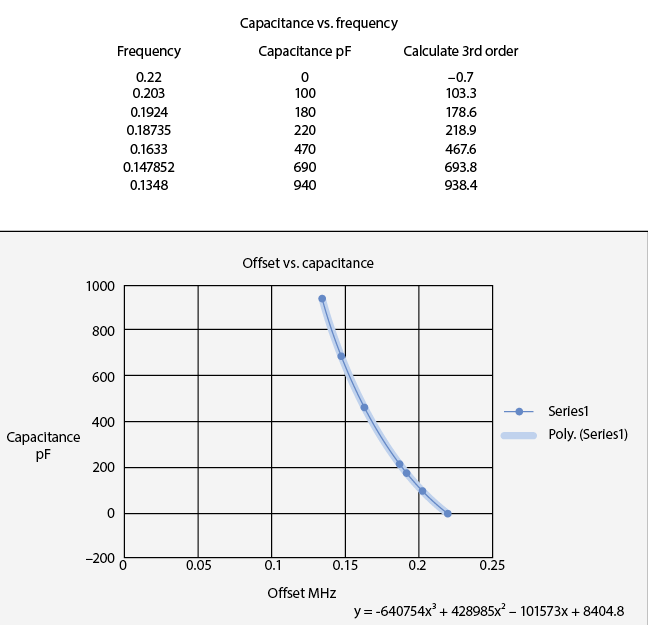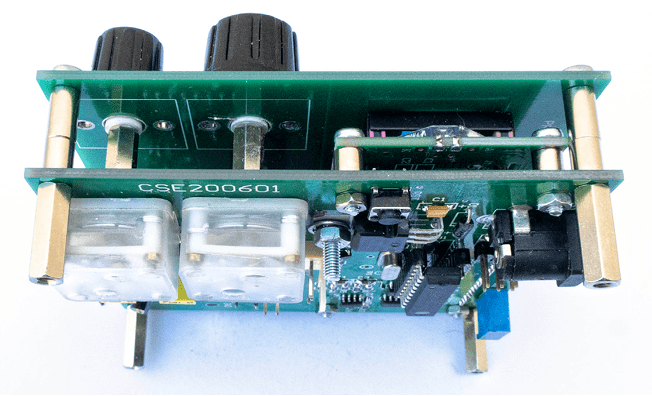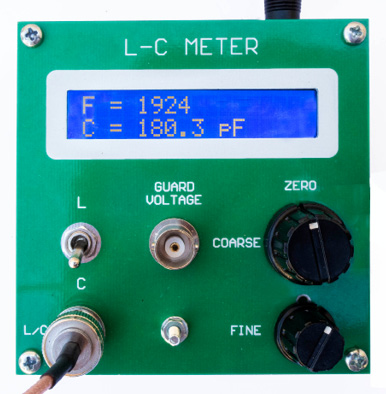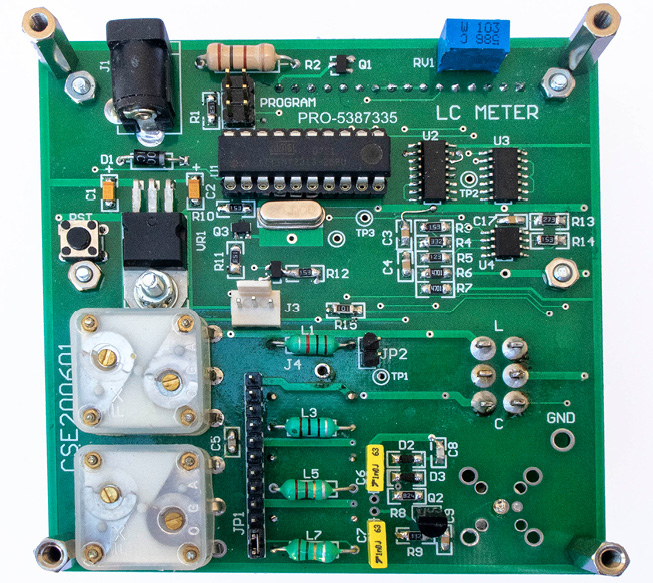# Building an LC MeterWritten by

## Retro Design Reimagined

### After reading about a restoration project on an old Tektronix L-C meter, Charles realized that the device is a brilliant design given the limitations of the era. That inspired him to embark on a similar design but using modern techniques and technologies.

• How to build a retro L-C meter design using modern technology

• The circuit design

• The oscillator design

• Why the components were chosen

• How the L – C measurement math works

• How to develop the firmware

• How to do the calibration

• Microchip Technology ATtiny4313 MCU

• 74HC161 counter

• J310 or 2N5484 JFET

• 6×2 LCD module with LED backlighting

• Texas Instruments (TI) LMV722

•  Vishay capacitors

How do we measure capacitance or inductance? Numerous instruments are available to do this—the simplest of which is the “C” measurement on many digital multimeters. That and other similar tools generally fail in accurate measurement below 1,000pF. While doing some RF work, I needed to measure chip capacitors down to about 10pF, and none of the inexpensive multimeters available on eBay and elsewhere could do the job accurately.

The Australian magazine, Silicon Chip, recently published a two-part article on restoring a Tektronix T130 LC meter designed in the 1950s . This was an impressive feat of engineering at the time. It was all done with analog techniques. The Tektronix design had a reference oscillator at 140kHz, FREF. The measurement oscillator was tuned to the same frequency, initially FTEST. Then, by placing a capacitor across the tuned circuit, or an inductor in series with the inductor in the oscillator, the test oscillator became lower in frequency.

By multiplying FREF with FTEST we get FREF + FTEST and FREF – FTEST. Selecting the latter by using a low-pass filter the Tektronix designers then used clever analog techniques to convert this to a capacitor or inductor value readout on a moving coil meter. Their design gave accurate measurements from 1pF to 300pF or 1µH to 300µH. It was an absolutely brilliant design, considering the limitations of what was available at the time.

I was so inspired that I decided to make a similar design, using modern techniques. What initially looked like a straightforward design proved, however, to have some interesting problems. But, as always, “the devil was in the details.”

Figure 1 is the schematic of the updated LC meter. I have an oscillator running at 220kHz, and a switch on the input that selects either the unknown capacitor value in parallel or the unknown inductor in series. This is amplified and applied to a Schmitt trigger to produce a square wave. The Microchip Technology ATtiny4313 microcontroller (MCU)  is not fast enough to count such a high frequency, so I have a divide-by-2 counter following the oscillator in the 74HC161 counter. I count the number of pulses in 0.5 seconds, which gives two readings per second. This, combined with the divide-by-2 counter, gives a readout of 1/10 the frequency. Whereas the Tektronix design compared the frequency with a reference oscillator, in this design, the frequency is measured directly. The readout is a 16×2 LCD module with LED backlighting, which is the most popular size for many projects.

##### OSCILLATOR DESIGN

I used a version of the Colpitts circuit for the oscillator. The J310 or 2N5484 JFET has been around for decades, but is still available and is an excellent choice for this oscillator. It is inherently stable, and gives a clean sinewave output as shown in Figure 2 and Figure 3. The diodes from gate to ground of the J-FET limit the output of just over 1V peak to peak over a wide range of frequencies. The two 1,000pF capacitors should be polystyrene dielectric for best stability, but good quality polyester appears to work well. Do not use ceramics.

The inductor in the oscillator consists of seven separate coils. This allows the actual inductance to be set to a value close to 1,000µH. Shorting links on each are used to adjust the total value. In my initial measuring of a batch of inductors, I found that the nominal 1,000µH was at least 5% low, hence the need to add extra ones in series. This is explained in the calibration procedure. The effective capacitance across the coil is made up of the two 1,000pF capacitors in series, and in parallel with the tuning capacitors plus strays.

How many variable capacitors can you easily buy? Once upon a time, they were readily available in many sizes, and if you scour eBay and elsewhere, a few are still available at ridiculously high prices. I have a few of these (shown in Figure 4), and while they are beautifully made and may be useful for a one-off design, they certainly can’t be specified for a new design. In fact, they are now really collectors’ items! It may be possible to use varicaps, but I was reluctant to use these because they would vary markedly in capacitance with the voltage swing across the tuned circuit, with possible undesirable results.

Fortunately, an easily obtainable type of variable capacitor is used for transistor radios (Figure 5). It has two sections, 6pF to 60pF and 6pF to 160pF, is available from multiple eBay sources and is reasonably priced. I used two of these, one with both sections in parallel for the coarse tuning, giving a range of 12pF to 220pF. Fine tuning is done by a 22pF capacitor connected to the 66pF section of the other variable capacitor, which gives it a range of about 5pF to 16pF.

##### COMPONENT CHOICES

Amplifier and frequency divider: The output at the JFET oscillator source is about 700mV peak to peak. This is fed into an op amp with a gain of 6, to produce a sine wave of 4.2VPP feeding into the 74HC14 Schmitt trigger. Various op amps are suitable. They need to have a rail-to-rail output with a single 5V supply, and have a gain bandwidth of at least 5MHz. Suitable ones are Analog Devices AD8606 or Texas Instruments (TI) LMV722. The output of the 74HC14 is a square wave feeding the clock input of a 74HC161 counter. In the early stages of the design, I was not sure what frequency oscillator I would use, and what division would be needed, hence the 74HC161 was chosen. As it turns out, only the divide-by-2 was used.

The other half of the op amp is set to a gain of about 1.7, so that the output is a close match to the 1V peak-to-peak of the tank circuit. It’s equivalent to the Guard Voltage of the Tektronix design. This was useful in measuring inter-electrode capacitance of vacuum tubes. I included it for completeness, but it is really of limited use, and may be safely omitted. It is, however, a convenient test point to measure the oscillator frequency and waveform.

MCU and display: The AVR ATtiny4313 was chosen because it has sufficient pins and memory for the job. The LCD module is probably the most popular type used with a 16×2 display and backlighting. There is a simplified RS232 interface included for debugging, which is set to 38400, n, 8, 1.

Power supply: This is the classical simple design using the venerable 7805 regulator. Input voltage is from a 9V or 12V regulated plugpack. A series diode is included for polarity protection. Current consumption is about 70mA. I did not bother with a power switch.

##### MEASUREMENT OF L OR C

The divide-by-2 counter means that for zero L or C added, adjust the coarse and fine capacitors so that 110kHz maximum is fed into the MCU. The top line of the display will show Offset = 0 when “zeroed.” If a capacitor in parallel with the tank circuit, or an inductor in series is added, both will result in a lower frequency.

C= 1/ω2L and L= 1/ω2C
where ω = 2πf

For the reference frequency of 220kHz, and the (nominal) 1,000µH inductor, the capacitor for the tuned circuit is 523pF. From this it would seem that if we measure the frequency and know these values, the L or C value can be readily calculated. This was what I initially did, only to find that the readings were woefully inaccurate. Why? The calculation assumes that the reference inductance value is constant. However, ferrites have a permeability that varies substantially over frequency, and hence, the lousy results. Table 1 shows the measurements I have made on a nominal 1,000µH ferrite inductor using a Q meter.

The Q is satisfactory over the entire range; however, the calculated inductance varies substantially. Using an air-cored inductor would give a constant inductance over the frequency range, but its size would be prohibitively large.

My initial idea was to modify the calculations according to the measured inductance, but I decided on a different approach. By measuring the frequency for a series of known capacitor values and plotting a capacitance against frequency curve, I could use this to calculate the unknown value. I bought a selection of 1% Vishay capacitors. Then, using Excel, I plotted this curve offset from 220kHz, and used the polynomial function in Excel that was a close approximation to the curve (Figure 6). It turns out that a second-order polynomial was inaccurate, but a third-order one represented the curve accurately. Figure 6 shows the Excel calculation that gives the equation:Figure 6 Using Excel, I plotted a curve of capacitance vs. frequency offset from 220kHz, and used the polynomial function in Excel that was a close approximation to the curve.
##### SNEAKY MATH

The calculations look rather daunting, and double precision floating point may be possible, but I wanted to use 32-bit integer arithmetic. The Δf in MHz had to be multiplied to get rid of the decimal point. Because this can result in astronomical numbers way beyond the 32-bit limit of 4,294,967,296, it required some sneaky arithmetic to maintain accuracy.

I opened a half-second window for counting the frequency pulses. Referring to Figure 1, the measured frequency is applied to a counter, and the divide-by-2 output is used. This means that the output of the counter is actually a quarter of the frequency. The measured value is then multiplied by 4 and divided by 25. This means that the number is now 1/100 of the actual frequency. You may think that this will reduce the resolution, but the effect is minimal. Dropping off the last two zeros of 220,000 will still give a resolution of 1 in 2,200.

The measured value is now subtracted from 2,200 to give the offset. This is the number that is placed on the top line of the LCD. With no external capacitor, tune this to become zero. With the capacitor in place, the frequency will drop. Let’s choose an example offset value of 325. Looking at the first term, the calculation gives a value way beyond 32 bits. Therefore, we use a sequence of multiplying and dividing. Listing 1 is the section of BASCOM code that does the capacitance calculation and shows how overflow is avoided.

``````Listing 1
This section of the BASCOM code does the capacitance calculation and shows how overflow is avoided.

‘ Calculate positive terms first

Capacitance = 84048                     ‘ this is the last term times 10
‘ to give 0.1 pF resolution

Temp_1 = Frequency * 428985             ‘ multiply and divide in sequence
Temp_1 = Temp_1 / 1000                  ‘ to avoid overflow of 32 bits
Temp_1 = Temp_1 * Frequency
Temp_1 = Temp_1 / 10000
Capacitance = Capacitance + Temp_1      ‘ add second term

Temp_1 = Frequency * 640754             ‘ Now calculate the first term
Temp_1 = Temp_1 / 1000
Temp_1 = Temp_1 * Frequency
Temp_1 = Temp_1 / 10000
Temp_1 = Temp_1 * Frequency
Temp_1 = Temp_1 / 10000

Capacitance = Capacitance - Temp_1      ‘ subtract first term

Temp_1 = Frequency * 101573             ‘ Calculate third term
Temp_1 = Temp_1 / 1000

Capacitance = Capacitance - Temp_1      ‘ subtract third term

Integers = Capacitance / 10             ‘ get the integer value
Fraction = Capacitance Mod 10
If Integers > 500 Then Lcd “C = “ ; Integers ; “ pF       “
If Integers =< 500 Then Lcd “C = “ ; Integers ; “.” ; Fraction ; “ pF        “``````

This may seem rather confusing with all the multiplying and dividing, but if you work your way through the code, the final number that comes out will give you a capacitance value of 217.7pF, which is what you get with a nominal 220pF capacitor. There is not much point maintaining a resolution of 0.1pF for larger values. The accuracy is not there, so anything greater than 500pF is truncated to just the integer value. Inductance calculations are not quite as accurate, and a second-order polynomial is adequate.

##### CONSTRUCTION

The LC meter has a “sandwich” construction (Figure 7). The front panel is a circuit board with all the required holes cut out (Figure 8). The middle section contains all the circuitry, and the LCD module is attached by M2.5×12 screws with 6mm spacers. The component side of the middle section is shown in Figure 9. The bottom plate could be a piece of 1.6mm aluminum or a piece of blank circuit board the same size as the front panel. The whole assembly is held together by M3 screws and spacers.

The knob that comes with the tuning capacitors is unsuitable. I replaced it with a 6.2mm (1/4″) diameter spacer, 25mm long, attached to the rotor by an M2.5×30 screw. Any suitable knob may then be used, as shown in Figure 8.

To minimize coupling between the tuning inductors, they are placed alternately on opposite sides of the circuit board. The underside of the board is mostly a ground plane, and that further shields the inductors from each other. Several capacitors are also placed on the underside of the board.

The BNC connector on the front panel is not convenient for connecting the unknown values of L and C. I designed a small PCB as shown in Figure 10. This connects by a short SMA-to-BNC cable to the LC meter. It has pads available for placement of 0805- or 1206-size SMDs (surface-mount devices). By holding these down carefully with a non-metallic stylus, the value can be measured. It’s a bit fiddly but does work.Figure 7 The LC meter has a “sandwich” construction. The whole assembly is held together by M3 screws and spacers.Figure 8 The front panel is a circuit board with all the required holes cut out. A readout for a 180pF capacitor is shown.Figure 9 The middle section contains all the circuitry, and the LCD module is attached by M2.5×12 screws with 6mm spacers. Shown here is the component side of the middle section.Figure 10 I designed this small PCB that connects by a short SMA-to-BNC cable to the LC meter.
##### FIRMWARE

The firmware is written in BASCOM, and the source code is available at Circuit Cellar’s article code and files download page for readers that may wish to modify it. The top line of the LCD always shows the offset from the original 220kHz frequency. The bottom line shows the L or C value.

Below 500pF, it shows the decimal value, for example, 46.7pF. For higher values, only the integer number will be shown. Capacitors can be read up to about 1,500pF, and inductors up to about 1,000µH. Higher values stop the oscillator from working.

To measure capacitance, set the toggle switch to “C”. Connect the measurement fixture and adjust the coarse and fine adjustments to read “Offset=0” on the top line. Connect the unknown, and the lower line will show the value.

To measure inductance, first set the switch to “C” and adjust to 0 as above. Then, set the switch to “L” and connect the unknown inductance. The lower line will show the inductance, but the accuracy is not as high as for capacitance, and only the whole number is shown. Also, values below 3µH cannot be measured with any accuracy. In these cases, the lower line will show “Too Low.”

##### CALIBRATION PROCEDURE

Because of the tolerance of the inductors used, it is necessary to calibrate to a known value of capacitance. Capacitors with 1% tolerance are available, and the Vishay brand can be bought at element14 (www.farnell.com) . I bought capacitors with several different values including 100pF, 220pF, 470pF and 2,200pF.

Start by shorting out all L2 to L7 inductors, using jumpers in JP1, leaving just the nominal 1,000µH coil, L1. Measure the 470pF capacitor, which will show a lower value. Remove jumpers one by one, and re-measure the capacitor until it reads close to the 470pF. It may require some trial and error to reach the correct value. Fortunately, there is no additional calibration is required for inductance. Testing the meter with the reference capacitors produced the results that are listed in Table 2.

Measurement of inductors is problematic. And as mentioned before, the inductance of ferrites varies substantially over frequency. I also used the Q-meter to calculate the inductances, though not at the same frequencies. In both cases, the measured values were way off the nominal value (Table 3). For example, the 470µH resonated with 670pF at 320kHz, which gave a calculated value of 369µH, while the measured value was 397µH!Table 3 The Q-meter was used to calculate the inductances, though not at the same frequencies. The measured values were way off the nominal value.

In conclusion, this LC meter project was a most interesting exercise in using the same philosophy that was applied to the 1950s Tektronix design. If nothing else, the experience highlights just how good the original design was.RESOURCES

References:
 Alan Hampel, Vintage Workbench: The Tektronix Type 130 LC Meter, Parts 1 and 2. Silicon Chip, June, 2020 and July, 2020. https://www.siliconchip.com.au/Issue/2020/June/Vintage+Workbench
https://www.siliconchip.com.au/Issue/2020/July/Vintage+Workbench
 ATtiny4313 www.microchip.com/en-us/product/ATtiny4313
 Vishay capacitors https://www.newark.com/c/passive-components/capacitors?brand=vishay

Analog Devices | www.analog.com
Farnell, an Avent Company | www.farnell.com
Microchip Technology | www.microchip.com
Tektronix | www.tek.com
Texas Instruments | www.ti.com
Vishay | www.vishay.com

PUBLISHED IN CIRCUIT CELLAR MAGAZINE • NOVEMBER 2021 #376 – Get a PDF of the issue

 Keep up-to-date with our FREE Weekly Newsletter! Don't miss out on upcoming issues of Circuit Cellar. Subscribe to Circuit Cellar Magazine Note: We’ve made the May 2020 issue of Circuit Cellar available as a free sample issue. In it, you’ll find a rich variety of the kinds of articles and information that exemplify a typical issue of the current magazine. Would you like to write for Circuit Cellar? We are always accepting articles/posts from the technical community. Get in touch with us and let's discuss your ideas.